# R S Aggarwal Solutions for Class 10 Maths Exercise 18C Chapter 18 Mean, Median, Mode of Grouped Data, Cumulative Frequency Graph and Ogive

R S Aggarwal Solutions for Class 10 Maths exercise 18C is available here. All exercise questions are answered in detail by the subject expert. This exercise is about ???. You can download Class 10 Maths Chapter 18 R S Aggarwal Solutions and clear your doubts on various concepts in class 10 Maths.

## Download PDF of R S Aggarwal Solutions for Class 10 Maths Chapter 18 Mean, Median, Mode of Grouped Data, Cumulative Frequency Graph and Ogive Exercise 18C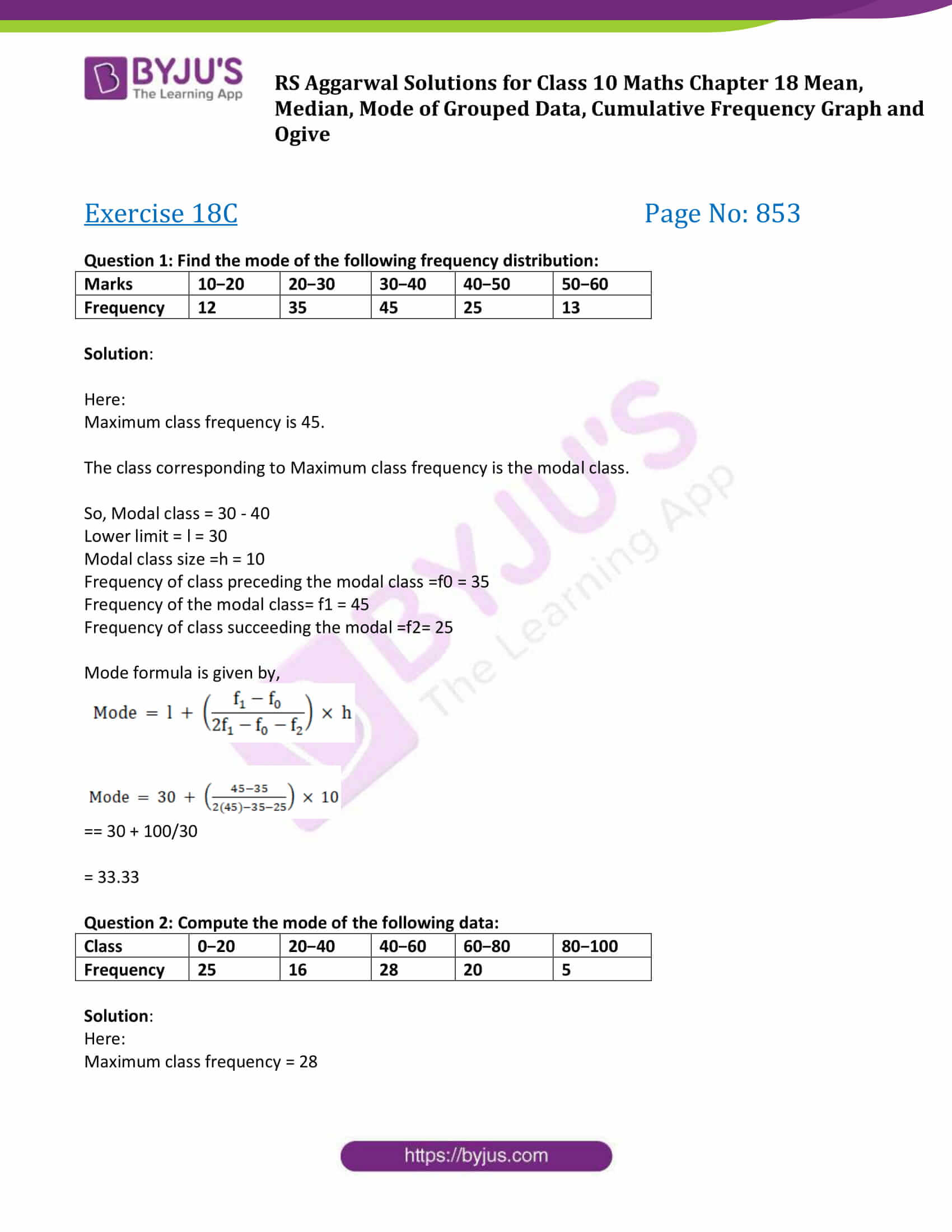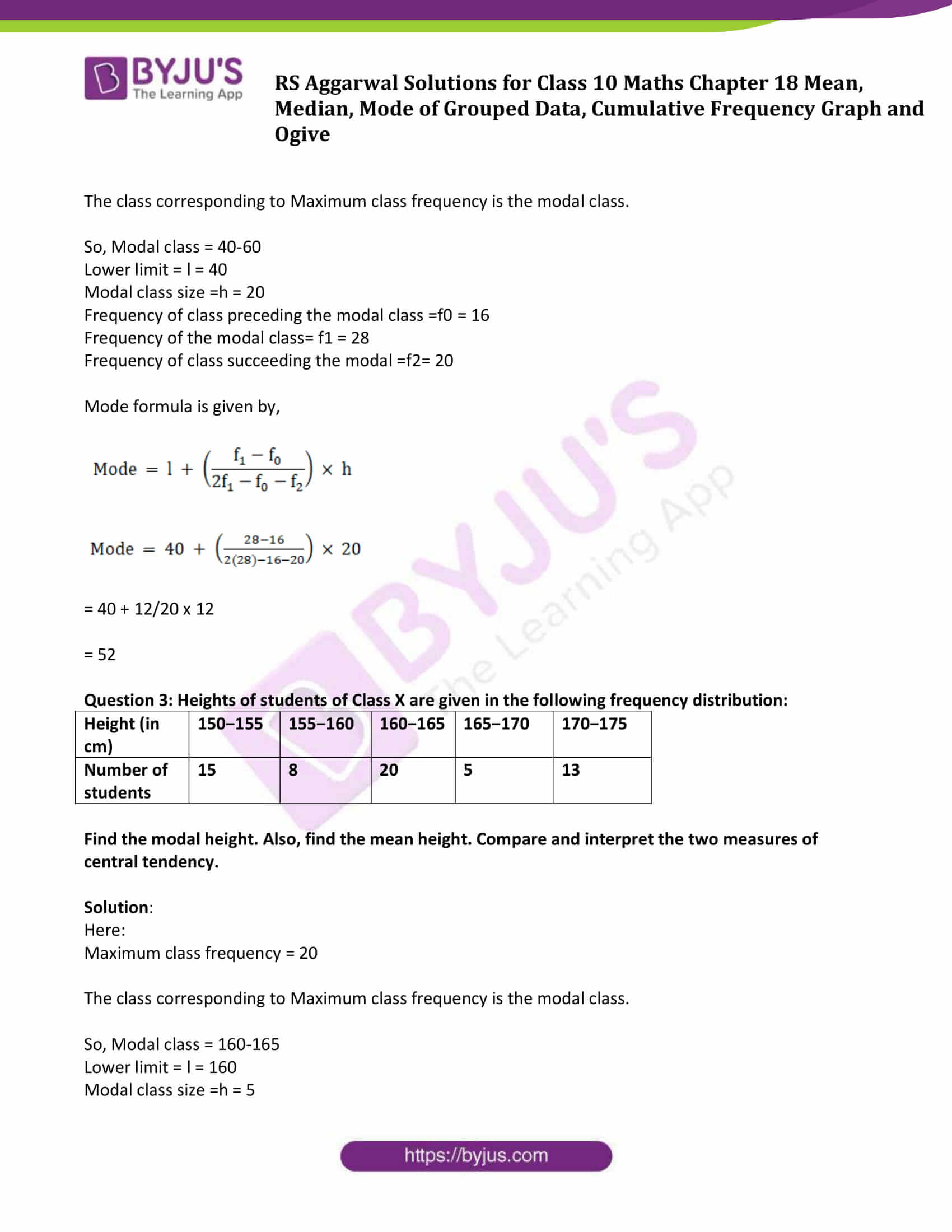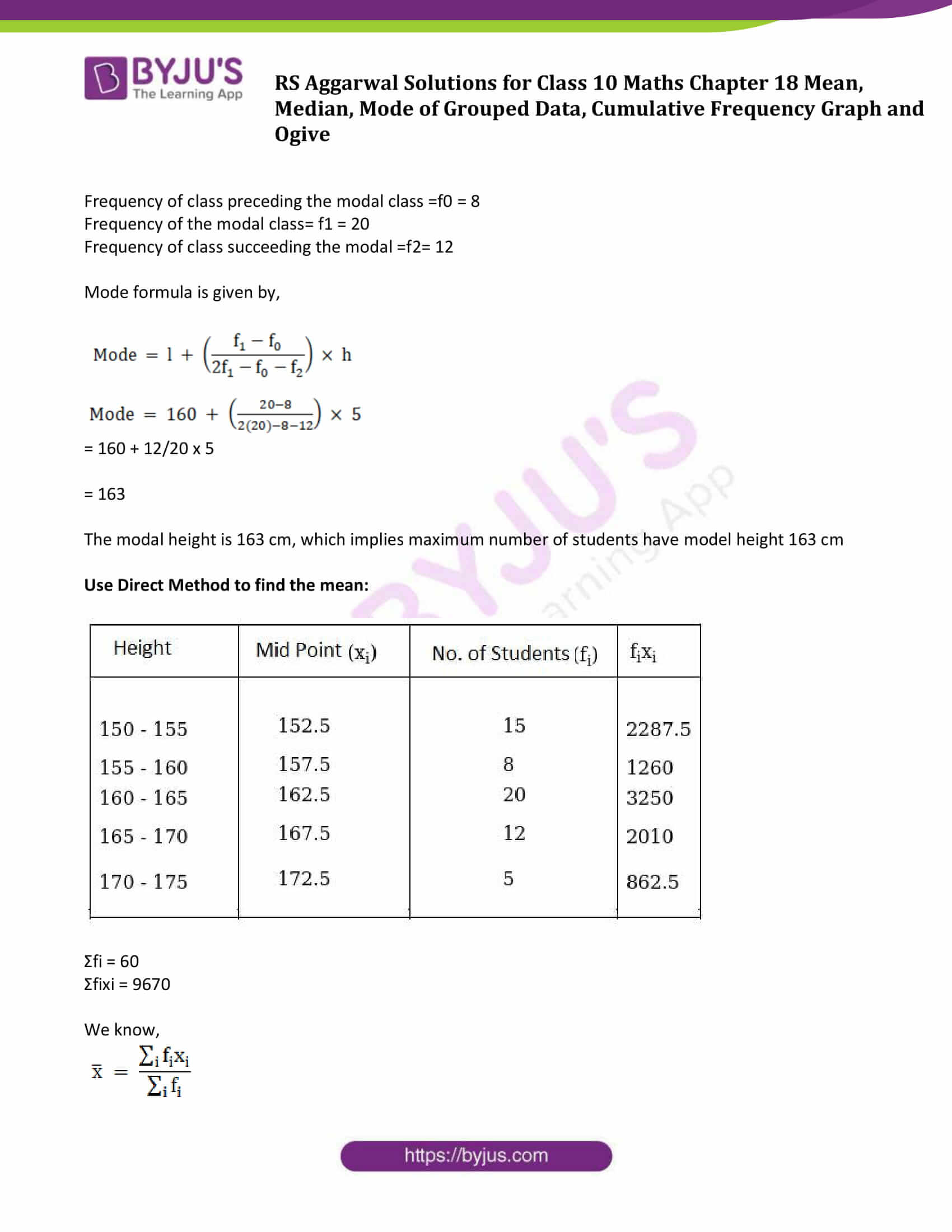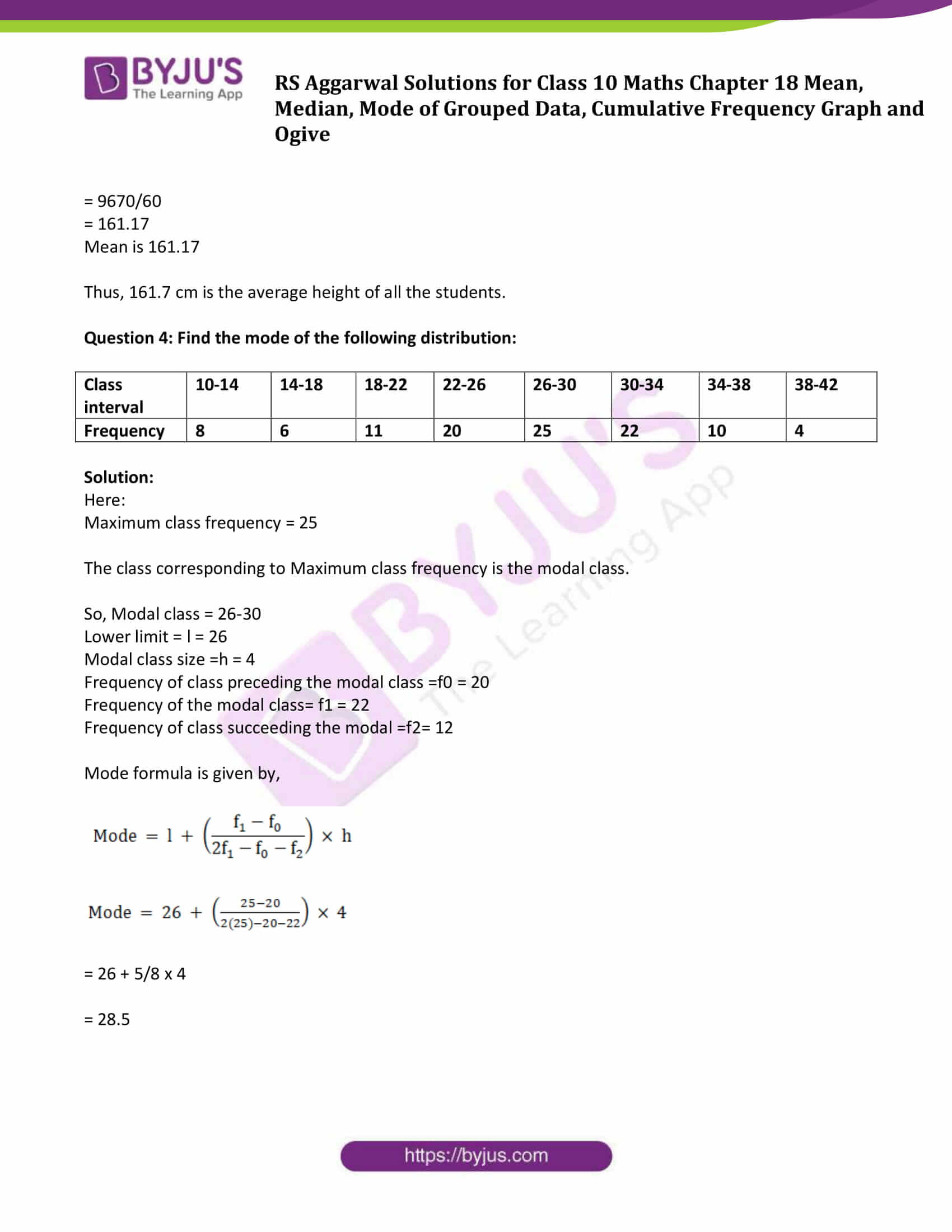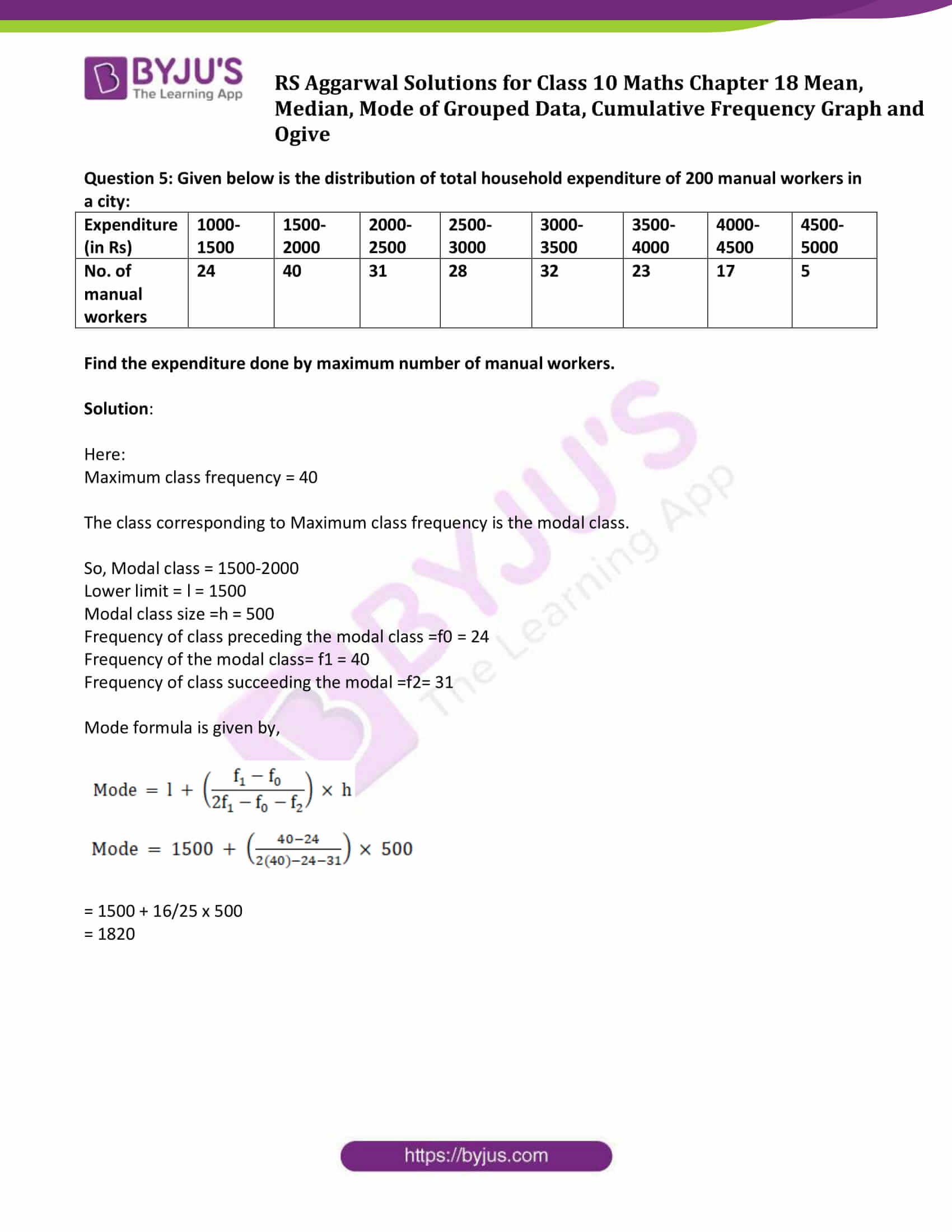## Access other exercise solutions of Class 10 Maths Chapter 18 Mean, Median, Mode of Grouped Data, Cumulative Frequency Graph and Ogive

Exercise 18 A Solutions

Exercise 18 B Solutions

Exercise 18 D Solutions

Exercise 18 E Solutions

## Exercise 18C

Question 1: Find the mode of the following frequency distribution:

 Marks 10−20 20−30 30−40 40−50 50−60 Frequency 12 35 45 25 13

Solution:

Here:

Maximum class frequency is 45.

The class corresponding to Maximum class frequency is the modal class.

So, Modal class = 30 – 40

Lower limit = l = 30

Modal class size =h = 10

Frequency of class preceding the modal class =f0 = 35

Frequency of the modal class= f1 = 45

Frequency of class succeeding the modal =f2= 25

Mode formula is given by,

$Mode = I +\left ( \frac{f_{1}-f_{0}}{2f_{1}-f_{0}-f_{2}} \right )\times h$ $Mode = 30 +\left ( \frac{45-35}{2\times 45-35-25} \right )\times 10$

= 30 + 100/30

= 33.33

Question 2: Compute the mode of the following data:

 Class 0−20 20−40 40−60 60−80 80−100 Frequency 25 16 28 20 5

Solution:

Here:

Maximum class frequency = 28

The class corresponding to Maximum class frequency is the modal class.

So, Modal class = 40-60

Lower limit = l = 40

Modal class size =h = 20

Frequency of class preceding the modal class =f0 = 16

Frequency of the modal class= f1 = 28

Frequency of class succeeding the modal =f2= 20

Mode formula is given by,

$Mode = I +\left ( \frac{f_{1}-f_{0}}{2f_{1}-f_{0}-f_{2}} \right )\times h$ $Mode = 40 +\left ( \frac{28-16}{2\times 28-16-20} \right )\times 20$

= 40 + 12/20 x 12

= 52

Question 3: Heights of students of Class X are given in the following frequency distribution:

 Height (in cm) 150−155 155−160 160−165 165−170 170−175 Number of students 15 8 20 5 13

Find the modal height. Also, find the mean height. Compare and interpret the two measures of central tendency.

Solution:

Here:

Maximum class frequency = 20

The class corresponding to Maximum class frequency is the modal class.

So, Modal class = 160-165

Lower limit = l = 160

Modal class size =h = 5

Frequency of class preceding the modal class =f0 = 8

Frequency of the modal class= f1 = 20

Frequency of class succeeding the modal =f2= 12

Mode formula is given by,

$Mode = I +\left ( \frac{f_{1}-f_{0}}{2f_{1}-f_{0}-f_{2}} \right )\times h$ $Mode = 160 +\left ( \frac{20-8}{2\times 20-8-12} \right )\times 5$

= 160 + 12/20 x 5

= 163

The modal height is 163 cm, which implies maximum number of students have model height 163 cm

Use Direct Method to find the mean: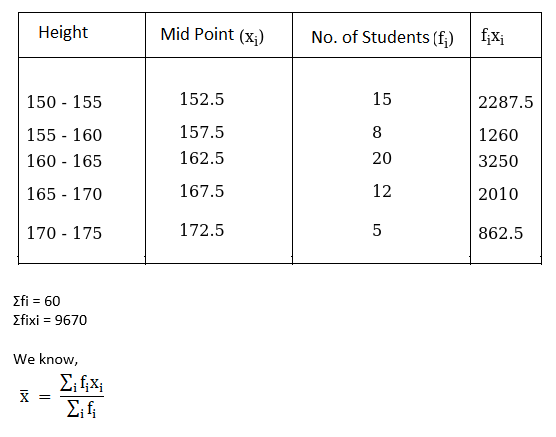= 9670/60

= 161.17

Mean is 161.17

Thus, 161.7 cm is the average height of all the students.

Question 4: Find the mode of the following distribution:

 Class interval 10-14 14-18 18-22 22-26 26-30 30-34 34-38 38-42 Frequency 8 6 11 20 25 22 10 4

Solution:

Here:

Maximum class frequency = 25

The class corresponding to Maximum class frequency is the modal class.

So, Modal class = 26-30

Lower limit = l = 26

Modal class size =h = 4

Frequency of class preceding the modal class =f0 = 20

Frequency of the modal class= f1 = 22

Frequency of class succeeding the modal =f2= 12

Mode formula is given by,

$Mode = I +\left ( \frac{f_{1}-f_{0}}{2f_{1}-f_{0}-f_{2}} \right )\times h$ $Mode = 26 +\left ( \frac{25-20}{2\times 25-20-22} \right )\times 4$

= 26 + 5/8 x 4

= 28.5

Question 5: Given below is the distribution of total household expenditure of 200 manual workers in a city:

 Expenditure (in Rs) 1000-1500 1500-2000 2000-2500 2500-3000 3000-3500 3500-4000 4000-4500 4500-5000 No. of manual workers 24 40 31 28 32 23 17 5

Find the expenditure done by maximum number of manual workers.

Solution:

Here:

Maximum class frequency = 40

The class corresponding to Maximum class frequency is the modal class.

So, Modal class = 1500-2000

Lower limit = l = 1500

Modal class size =h = 500

Frequency of class preceding the modal class =f0 = 24

Frequency of the modal class= f1 = 40

Frequency of class succeeding the modal =f2= 31

Mode formula is given by,

$Mode = I +\left ( \frac{f_{1}-f_{0}}{2f_{1}-f_{0}-f_{2}} \right )\times h$ $Mode = 1500 +\left ( \frac{40-24}{2\times 40-24-31} \right )\times 10$

= 1500 + 16/25 x 500

= 1820

Class 10 Maths Chapter 18 Mean, Median, Mode of Grouped Data, Cumulative Frequency Graph and Ogive Exercise 18C is based on Mode of Grouped Data.